# The stochastic volatility model

The stochastic volatility model, introduced by Taylor (1982), is defined by \begin{equation} \begin{aligned} y_t &= \sigma_y e^{h_t/2} \epsilon_t, \quad t = 1, \dots, T, \\ h_{t+1} &= \phi h_{t} + \sigma_h \eta_t, \quad t = 1, \dots, T-1, \\ \eta_t &\stackrel{\text{iid}}{\sim} \mathcal{N}(0,1), \\ \epsilon_t &\stackrel{\text{iid}} {\sim} F \end{aligned} \end{equation} where $$y_t$$ is the observed log returns, $$h_t$$ is the logarithm of the variance on day $$t$$. The distribution of the innovations $$\epsilon_t$$ is specified below. To ensure stationarity for $$h_t$$, we assume $$|\phi| < 1$$. It can be shown that the unconditional distribution of $$h_t$$ is $$\mathcal{N}(0,\sigma_h^2/(1 - \phi^2))$$, and we assume $$h_1 \sim \mathcal{N}(0,\sigma_h^2/(1-\phi^2))$$. An interpretation of the latent process $$\{h_t\}$$ is that is represents the random and uneven flow of new information into the marked. For different time points, the variance will be dependent of this unobserved flow’’ of information, i.e. conditioning on $$h_t$$, $$\mathrm{Var}(y_t | h_t) = \sigma_x^2 e^{h_t}$$.

The original SV model from Taylor (1982) assumed normally distributed innovations, but this is usually to strong of an assumption. Financial returns are usually heavy-tailed, might be asymmetric and the volatility can be correlated with the returns. The latter is called the leverage effect, where there is a negative correlation between a change in price and the volatility, meaning that a drop in price tend to lead to an increase in volatility To take these features of financial time series into account stochvolTMB has implemented the following four distribution for the innovations:

• Gaussian: $$\epsilon_t \sim \mathcal{N}(0,1)$$
• t: $$\epsilon_t \sim t_\nu(0,1)$$, where $$\nu$$ is the degrees of freedom.
• Skew-Gaussian: $$\epsilon_t \sim \mathcal{SN}(0,1, \alpha)$$, with zero mean, unit variance and skewness parameter $$\alpha$$.
• Leverage: $$\epsilon_t \sim \mathcal{N}(0,1)$$ and $$\mathrm{Cor}(\epsilon_t, \eta_t) = \rho$$

stochvolTMB is inspired by the package stochvol (Kastner (2016)), but stochvolTMB obtain parameter estimates through maximum likelihood estimation and not Markov Chain Monte Carlo.

# Usage

The main functions of stochvolTMB are:

Function Name Description
estimate_parameters Estimate parameters of a stochastic volatility model.
sim_sv Simulate data from a stochastic volatility model.
plot.stochvolTMB Plot estimated volatility and predicted (with confidence intervals).
summary.stochvolTMB Extract parameter estimates and volatility with uncertainty.
predict.stochvolTMB Predict future volatility and returns.

estimate_parameters returns an object of class stochvolTMB. The summary function returns a data.table with estimated parameters, estimated log-volatility and transformed parameters along with standard errors, p-values and z-values. The argument report = "fixed" returns the parameters on the scale they were estimated. This means that the standard deviations $$\sigma_y, \sigma_h$$ are given on the log scale; the degrees of freedom is one the scale $$\log (\nu - 2)$$ to ensure that $$\nu > 2$$ (i.e. the variance exists); and lastly that the persistence parameter $$\phi$$ and the correlation parameter $$\rho$$ are estimated on a logit scale to ensure that they are between -1 and 1. If report = c("fixed", "transformed"), parameter estimates transformed back to their original scale is also returned. If you want to extract the estimated log-volatility you can use report = "random".

# Example

Estimating parameters in a SV model is easy with stochvolTMB. As an example we investigate the daily log-returns of the S&P500 from 2005 to 2018. A quick look at the data:

data(spy)
plot(spy$date, spy$log_return, type = "l", xlab = "", ylab = "", main = "Log-returns of S&P500")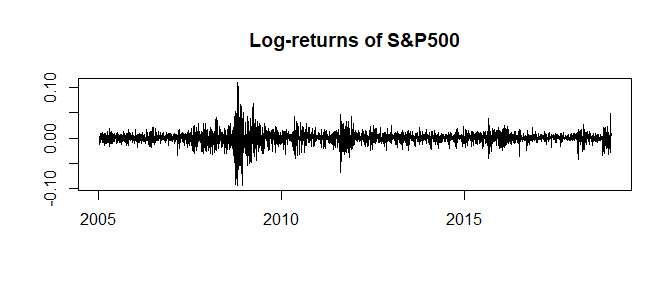plot(spy$date, spy$price, type = "l", xlab = "", ylab = "", main = "Price of S&P500")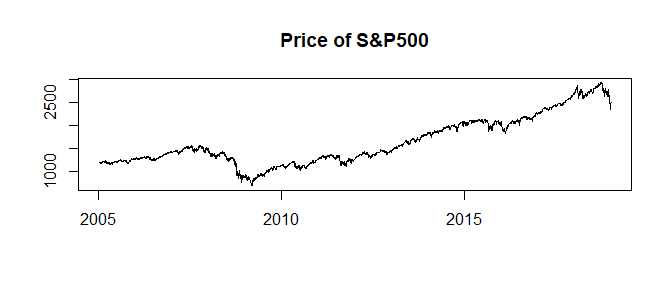We fit all four distributions:

gaussian = estimate_parameters(spy$log_return, model = "gaussian", silent = TRUE) t_dist = estimate_parameters(spy$log_return, model = "t", silent = TRUE)
skew_gaussian = estimate_parameters(spy$log_return, model = "skew_gaussian", silent = TRUE) leverage = estimate_parameters(spy$log_return, model = "leverage", silent = TRUE)

We can investigate the estimate for the degrees of freedom (df) to see if the returns are heavy-tailed

summary(t_dist, report = "transformed")
#>    parameter     estimate    std_error    z_value      p_value        type
#> 1:   sigma_y  0.008392879 0.0008689248   9.658924 4.505714e-22 transformed
#> 2:   sigma_h  0.185766767 0.0182175649  10.197124 2.042308e-24 transformed
#> 3:       phi  0.984924644 0.0039281816 250.732972 0.000000e+00 transformed
#> 4:        df 10.086366735 2.1022434966   4.797906 1.603330e-06 transformed

Clearly the returns are more heavy tailed than Gaussian, even when controlling for the stochastic volatility. We can also check for asymmetric returns

summary(skew_gaussian, report = "fixed")
#>      parameter  estimate  std_error    z_value      p_value  type
#> 1: log_sigma_y -4.797828 0.09127165 -52.566466 0.000000e+00 fixed
#> 2: log_sigma_h -1.556355 0.08899553 -17.488015 1.768107e-68 fixed
#> 3:   logit_phi  4.623512 0.23363755  19.789252 3.684695e-87 fixed
#> 4:       alpha -1.088828 0.14275937  -7.627013 2.402559e-14 fixed

and leverage (rho)

summary(leverage, report = "transformed")
#>    parameter     estimate    std_error   z_value       p_value        type
#> 1:   sigma_y  0.008338412 0.0004163314  20.02830  3.121144e-89 transformed
#> 2:   sigma_h  0.273443559 0.0182641070  14.97164  1.125191e-50 transformed
#> 3:       phi  0.967721215 0.0043681868 221.53842  0.000000e+00 transformed
#> 4:       rho -0.748695259 0.0322487815 -23.21623 3.121690e-119 transformed

There is clear evidence for both asymmetric returns and a negative correlation (of -0.74!) between log-returns and the volatility. To find the model that fits the data best, we can compare the AIC of our models and pick the smallest.


AIC(gaussian,
t_dist,
skew_gaussian,
leverage)
#>               df       AIC
#> gaussian       3 -23430.57
#> t_dist         4 -23451.69
#> skew_gaussian  4 -23440.87
#> leverage       4 -23608.85

Clearly the leverage model outperforms the others and is our preferred model for this dataset. Lastly, we can also plot the estimated log-volatility and volatility:

plot(leverage, include_ci = TRUE, plot_log = TRUE, dates = spy$date)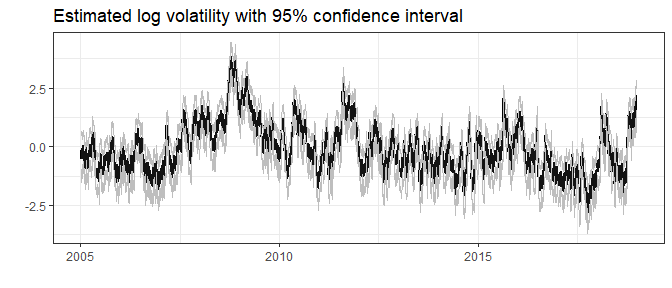plot(leverage, include_ci = TRUE, plot_log = FALSE, dates = spy$date)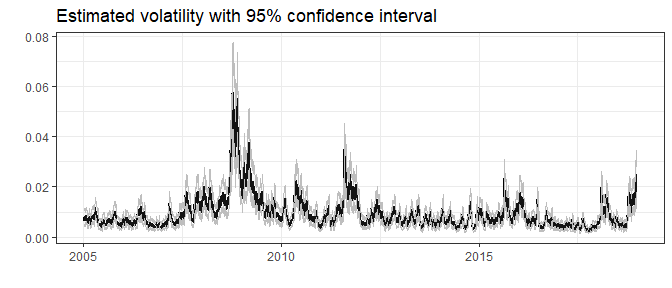We can simulate future returns with predict. This function returns three matrices of dimension $$\#$$ steps $$\times$$ #$simulations: (1) the latent log-volatility h; (2) one for the percentage volatility 100 * sigma_y * exp(0.5 * h) and (3) future returns. If the argument include_parameters is set to TRUE, the fixed parameters are simulated from their asymptotic multivariate distribution. This usually leads to broader uncertainty bands. To summarize the output from predict, we use the summary function, that calculate the mean and different quantiles based on the sumulations. We use the leverage model as an example:  pred = predict(leverage, steps = 10, include_parameters = TRUE) summary(pred) #>$y
#>     time quantile_0.025 quantile_0.975          mean
#>  1:    1    -0.03697127     0.03680939 -9.955825e-05
#>  2:    2    -0.03648798     0.03840502  1.568988e-04
#>  3:    3    -0.03730617     0.03762548  1.101025e-04
#>  4:    4    -0.03754075     0.03668388 -1.488216e-04
#>  5:    5    -0.03738328     0.03561644 -2.068317e-04
#>  6:    6    -0.03673173     0.03751472 -3.566262e-04
#>  7:    7    -0.03681117     0.03600734 -1.887601e-04
#>  8:    8    -0.03565664     0.03503671  5.997296e-05
#>  9:    9    -0.03554914     0.03611945  3.988628e-04
#> 10:   10    -0.03517953     0.03379153 -4.540136e-05
#>
#> $h #> time quantile_0.025 quantile_0.975 mean #> 1: 1 0.406543457 2.496289 1.452232 #> 2: 2 0.240709893 2.555045 1.404871 #> 3: 3 0.148866839 2.587771 1.359087 #> 4: 4 -0.007521566 2.602513 1.312440 #> 5: 5 -0.103075631 2.624392 1.273132 #> 6: 6 -0.220723063 2.659166 1.235431 #> 7: 7 -0.297637708 2.692436 1.199161 #> 8: 8 -0.391764255 2.712487 1.160312 #> 9: 9 -0.458938948 2.717130 1.124303 #> 10: 10 -0.573202451 2.724519 1.079538 #> #>$h_exp
#>     time quantile_0.025 quantile_0.975       mean
#>  1:    1    0.010133686     0.02928523 0.01790396
#>  2:    2    0.009362090     0.03009821 0.01758150
#>  3:    3    0.008854835     0.03063276 0.01732607
#>  4:    4    0.008231126     0.03099414 0.01703866
#>  5:    5    0.007801651     0.03150214 0.01673701
#>  6:    6    0.007537907     0.03199674 0.01659772
#>  7:    7    0.007156487     0.03236181 0.01638999
#>  8:    8    0.006906869     0.03250785 0.01614453
#>  9:    9    0.006583977     0.03235060 0.01584938
#> 10:   10    0.006211188     0.03258687 0.01558458

# plot the forecast
plot(leverage, forecast = 50) + ggplot2::xlim(3200, nrow(spy) + 50)
#> Warning: Removed 3199 row(s) containing missing values (geom_path).

#> Warning: Removed 3199 row(s) containing missing values (geom_path).

#> Warning: Removed 3199 row(s) containing missing values (geom_path).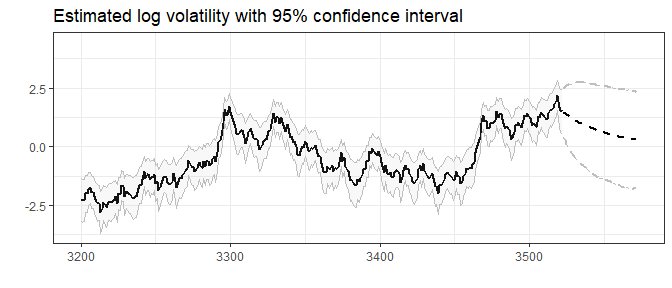# Comparison to stochvol

The R-package stochvol (Kastner (2016)) provides a Bayesian framework for inference using Markov Chain Monte Carlo. An advantage of stochvolTMB is that optimization can be a lot faster than MCMC. We here compare the leverage and the gaussian model. Depending on your machine you can expect a speed up of 20-200x.

library(stochvol)

stochvol_gauss <- svsample(spy$log_return, quiet = T) stochvolTMB_gauss <- estimate_parameters(spy$log_return, "gaussian", silent = TRUE)

stochvol_lev <- svlsample(spy$log_return, quiet = T) stochvolTMB_lev <- estimate_parameters(spy$log_return, "leverage", silent = TRUE)

We can compare the parameter estimates of the two methods. Note that the parameter exp(mu/2) and sigma from stochvol is the same as sigma_y and sigma_h from stochvolTMB. Both methods give almost identical results.


stochvol_gauss$para #> mean sd 5% 50% 95% #> mu -9.611533785 0.1854455626 -9.91175182 -9.611774497 -9.304678419 #> phi 0.978189757 0.0049148914 0.96968612 0.978414831 0.985836137 #> sigma 0.229775055 0.0203786934 0.19733459 0.228942708 0.264098047 #> exp(mu/2) 0.008217761 0.0007671236 0.00704191 0.008181439 0.009539261 #> sigma^2 0.053211825 0.0094502446 0.03894094 0.052414764 0.069747778 #> ESS #> mu 6230.1953 #> phi 327.9347 #> sigma 152.4001 #> exp(mu/2) 6230.1953 #> sigma^2 152.4001 summary(stochvolTMB_gauss, report = "transformed") #> parameter estimate std_error z_value p_value type #> 1: sigma_y 0.008185162 0.0007314791 11.18988 4.570586e-29 transformed #> 2: sigma_h 0.222440223 0.0190056672 11.70389 1.217431e-31 transformed #> 3: phi 0.979034243 0.0046595769 210.11226 0.000000e+00 transformed stochvol_lev$para
#>                   mean           sd           5%          50%          95%
#> mu        -9.569375399 0.1053851888 -9.739817718 -9.571446103 -9.400002030
#> phi        0.966952934 0.0041187166  0.960195391  0.966853189  0.973557574
#> sigma      0.276351629 0.0172514582  0.248100974  0.277044222  0.304057250
#> rho       -0.724949416 0.0328632332 -0.775144574 -0.726905055 -0.667578807
#> exp(mu/2)  0.008368349 0.0004417329  0.007674065  0.008348085  0.009095268
#> sigma^2    0.076667806 0.0095068932  0.061554093  0.076753501  0.092450811
#>                 ESS
#> mu        368.69311
#> phi       140.52279
#> sigma      42.20112
#> rho        26.56514
#> exp(mu/2) 368.69311
#> sigma^2    42.20112
summary(stochvolTMB_lev, report = "transformed")
#>    parameter     estimate    std_error   z_value       p_value        type
#> 1:   sigma_y  0.008338412 0.0004163314  20.02830  3.121144e-89 transformed
#> 2:   sigma_h  0.273443559 0.0182641070  14.97164  1.125191e-50 transformed
#> 3:       phi  0.967721215 0.0043681868 221.53842  0.000000e+00 transformed
#> 4:       rho -0.748695259 0.0322487815 -23.21623 3.121690e-119 transformed

# Estimation

The R-package TMB (Kristensen et al. (2016)) is used to implement our models for maximum likelihood estimation, since TMB lets us estimate parameters in models with a high number of latent variables.

Parameter estimation of stochastic volatility models is hard due to the fact the likelihood function is expressed as a high dimensional integral over the latent variables that cannot be evaluated analytically. If $$\boldsymbol{y} = (y_1, \ldots, y_T)$$ denotes our observations, $$\boldsymbol{h} = (h_1, \ldots, h_T)$$ our latent variables and $$\boldsymbol{\theta}$$ the parameters of interest, the likelihood of $$\boldsymbol{\theta}$$ is given by

$\begin{equation} \mathcal{L}(\boldsymbol{\theta}) = \int f_{\boldsymbol{y}}(\boldsymbol{y}|\boldsymbol{h})f_{\boldsymbol{h}}(\boldsymbol{h}) \, d\boldsymbol{h}, \end{equation}$

The conditional density of our observations given $$\boldsymbol{h}$$ is denoted by $$f_{\boldsymbol{y}}(\boldsymbol{y|u})$$, and $$f_{\boldsymbol{h}}(\boldsymbol{h})$$ denotes the marginal density of $$\boldsymbol{h}$$. To approximate this integral we apply the Laplace approximation.

## Laplace Approximation

Let $$\boldsymbol{y}$$ be a vector of observations, $$\boldsymbol{\theta}$$ our parameters of interest and $$\boldsymbol{h}$$ be a random vector of latent variables. Let $$g(\boldsymbol{h},\boldsymbol{\theta})$$ denote the negative joint log-likelihood. The likelihood of $$\boldsymbol{\theta}$$ is given by

$\begin{equation} \mathcal{L}(\boldsymbol{\theta}) = \int f(\boldsymbol{y},\boldsymbol{h}) \, d\boldsymbol{h} = \int f_{\boldsymbol{y}}(\boldsymbol{y|u}) f_{\boldsymbol{h}}(\boldsymbol{h}) \, d\boldsymbol{h} = \int \exp \{-g(\boldsymbol{h},\boldsymbol{\theta})\} \, d\boldsymbol{h}. \end{equation}$

We assume that $$g$$ has a global minimum at $$\boldsymbol{\hat{h}}$$ for a given $$\boldsymbol{\theta}$$, i.e. $$\boldsymbol{\hat{h}} = \text{argmin}_{\boldsymbol{h}} g(\boldsymbol{h},\boldsymbol{\theta})$$, and that $$g$$ is twice differentiable. The solution $$\hat{\boldsymbol{h}}$$ is known as the Empirical Bayes (EB) estimate. A second order Taylor expansion around $$\boldsymbol{\hat{h}}$$ yields

$\begin{equation} g(\boldsymbol{h},\boldsymbol{\theta}) \approx g(\boldsymbol{\hat{h}},\boldsymbol{\theta}) + \nabla g(\boldsymbol{\hat{h}},\boldsymbol{\theta})(\boldsymbol{h} - \boldsymbol{\hat{h}}) + \frac{1}{2}(\boldsymbol{h} - \boldsymbol{\hat{h}})^T\mathbb{H}(\boldsymbol{h} - \boldsymbol{\hat{h}}) \end{equation}$

Since $$\boldsymbol{\hat{h}}$$ is a minimum, $$\nabla g(\boldsymbol{\hat{h}},\boldsymbol{\theta}) = 0$$. Therefore

$\begin{equation} \mathcal{L}(\boldsymbol{\theta}) \approx \exp \{-g(\boldsymbol{\hat{h}},\boldsymbol{\theta})\} \int \exp \bigg \{-\frac{1}{2}(\boldsymbol{h} - \boldsymbol{\hat{h}})^T\mathbb{H}(\boldsymbol{h} - \boldsymbol{\hat{h}}) \bigg \} d\boldsymbol{h} \end{equation}$

We can observe that the integrand is the kernel of a multivariate normal density with covariance matrix $$\mathbb{H}^{-1}$$. The approximation is therefore given by

$\begin{equation} \mathcal{L}(\boldsymbol{\theta}) \approx \exp \{-g(\boldsymbol{\hat{h}},\boldsymbol{\theta})\} (2\pi)^{\text{dim}(\boldsymbol{h})/2} \text{det}(\mathbb{H})^{-1/2}, \end{equation}$

where we have used the fact that $$\text{det}(\mathbb{H}^{-1}) = \text{det}(\mathbb{H})^{-1}$$. The corresponding negative log-likelihood is

$\begin{equation} -l(\boldsymbol{\theta}) = -\frac{\text{dim}(\boldsymbol{h})}{2} \log (2\pi) + \frac{1}{2} \log \text{det}(\mathbb{H}) + g(\boldsymbol{\hat{h}},\boldsymbol{\theta}). \end{equation}$

Finding the optimal value of $$\boldsymbol{\theta}$$ can be viewed as a nested optimization problem. To find $$\boldsymbol{h} (\boldsymbol{\theta})$$ and $$\mathbb{H}(\boldsymbol{\theta})$$ we fix $$\boldsymbol{\theta}$$ and optimize using a quasi-Newton algorithm or a limited memory Newton method. The Laplace approximation is then optimized w.r.t. $$\boldsymbol{\theta}$$ using the quasi-Newton algorithm.